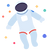### Gravity and Motion

#### Newton's Law of Universal Gravitation

###### Content ContributorsDev Lohar

# Learning Objectives###### ​###### Newton's Law of Universal GravitationNewton’s law of universal gravitation is essentially an equation. In words, the law states that any 2 bodies in the universe attract each other with a force that is directly proportional to the product of their masses and inversely proportional to the square of the distance between them. In simpler terms (hence an equation) it can be expressed as:###### ​This law means that any 2 bodies which have a mass, will attract each other. You and the bookshelf next to you attract each other with a gravitational force. But due to the very low mass of you and the bookshelf, and the low value of the gravitational constant, the force is next to nothing, hence you don’t feel anything. But since the mass of the Earth is 5.97 x 1024 kg, the gravitational force attracting you and the earth is much, much higher and hence you feel it. It’s what we call gravity. It’s the force attracting you and the Earth (which can be calculated using the equation above) that prevents you from floating out into space. One key thing to note about this is that the magnitude of the force experienced by each object is the same (due to Newton’s 3rd law; every action has an equal and opposite reaction). The magnitude of the force attracting the Earth towards you is the exact same magnitude of force that is attracting you towards the Earth.

So technically you can tell your friends that you are as strong as the Earth because you are applying the same force as the Earth is applying.

Now you might be wondering that if you are applying the same force as the Earth, then why is it that you fall towards the Earth but the Earth doesn’t fall towards you? This is because Newton’s 2nd law states that F = ma. The force is the same for both you and the Earth, but the mass of the Earth is significantly larger than yours. Hence, the acceleration for the Earth would have to be very small and your acceleration would have be high. Therefore, you accelerate faster towards the Earth but the Earth doesn’t accelerate towards you since its acceleration is very small that it becomes negligible.###### ​###### Apparent weightWeight is different to apparent weight. Scientifically, weight is the force of gravity, and apparent weight is the normal force you experience.

For example, when you are accelerating up on an elevator, you might feel heavier because your apparent weight has increased, whereas your (true) weight stays the same. Diagram 1 above should make it easier to understand. There is always the force of gravity (or weight force) acting downwards (red arrow). Since the elevator is accelerating up, there is a net force upwards (blue arrow). The net force can also be called the resultant force meaning it is the resulting force after 2 or more forces are acting on an object. Since the net force is upwards and weight force it downwards, it means the normal force (force that the floor is applying on you) has to be up; it balances the weight force and then provides the force to accelerate you upwards (hence the net force up). Therefore, making it larger than your weight force; making you feel heavier.

It is the same when accelerating down. The weight force stays the same; same magnitude and direction. The net force is now acting downwards because the elevator is accelerating downwards. So this means that the normal force has to be acting down but with a lower magnitude than the weight force; therefore making the net force down. See diagram 2.###### Apparent weightlessnessAs weight is different to apparent weight, weightlessness is also different to apparent weightlessness. When someone says, astronauts are weightless in the ISS (International Space Station), they are wrong. Nobody or nothing with a mass can be weightless because as we learnt earlier, weight is the force of gravity and no matter where you are, you’ll always experience the force of gravity from everything since gravity has an infinite range. Yes you might not feel the force, but it is always there, so it’s wrong to say the astronauts are weightless. The correct term is apparent weightlessness. This is when you or an object experiences 0 apparent weight or 0 normal force. However, apparent weightlessness is only possible when you are in free-fall.

Free fall is when you are constantly falling down. But since you are travelling horizontally at a velocity relative to the Earth and the curved surface of the Earth is constantly falling away from below you as the Earth rotates, the distance between you and the Earth’s surface remains the same. The resulting effect is that you fall in a curved path that almost matches the curvature of the Earth.

In terms of the astronauts, both the ISS and the astronauts are in free fall and so the astronauts do not experience a normal force, allowing them to experience apparent weightlessness.###### ​###### ​###### ​###### Inclined Planes#### Registrations Now Open for Empowered AcademyA Free Student-Centred Revision Program